# Lennard-Jones model

(diff) ← Older revision | Latest revision (diff) | Newer revision → (diff)

The Lennard-Jones intermolecular pair potential is a special case of the Mie potential and takes its name from Sir John Edward Lennard-Jones  . The Lennard-Jones model consists of two 'parts'; a steep repulsive term, and smoother attractive term, representing the London dispersion forces . The Lennard-Jones potential is probably the most extensively studied model potential today . Apart from being an important model in itself, the Lennard-Jones potential frequently forms one of 'building blocks' of many force fields and molecular-based equation of state models . It is worth mentioning that the 12-6 Lennard-Jones model is not the most faithful representation of the potential energy surface, but rather its use is widespread due to its computational expediency. For example, the repulsive term is maybe better described with the exp-6 potential. One of the first computer simulations using the Lennard-Jones model was undertaken by Wood and Parker in 1957  in a study of liquid argon. Thermophysical property data sampled by molecular simulations since then until 2019 has been compiled, digitalized and assessed by Stephan et al . This database contains about 35,000 datapoints.

## Functional form

The Lennard-Jones potential is given by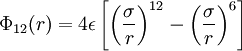$\Phi_{12}(r) = 4 \epsilon \left[ \left(\frac{\sigma}{r} \right)^{12}- \left( \frac{\sigma}{r}\right)^6 \right]$

or is sometimes expressed as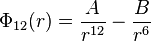$\Phi_{12}(r) = \frac{A}{r^{12}}- \frac{B}{r^6}$

where

•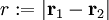$r := |\mathbf{r}_1 - \mathbf{r}_2|$
•$\Phi_{12}(r)$ is the intermolecular pair potential between two particles or sites
•$\sigma$ is the value of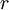$r$ at which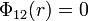$\Phi_{12}(r)=0$
•$\epsilon$ is the well depth (energy)
•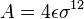$A= 4\epsilon \sigma^{12}$,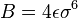$B= 4\epsilon \sigma^{6}$
• Minimum value of$\Phi_{12}(r)$ at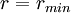$r = r_{min}$;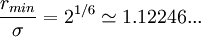$\frac{r_{min}}{\sigma} = 2^{1/6} \simeq 1.12246 ...$

In reduced units:

• Density: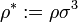$\rho^* := \rho \sigma^3$

where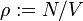$\rho := N/V$ (number of particles$N$ divided by the volume$V$)

• Temperature: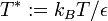$T^* := k_B T/\epsilon$

where$T$ is the absolute temperature and$k_B$ is the Boltzmann constant

The following is a plot of the Lennard-Jones model for the Rowley, Nicholson and Parsonage parameter set  (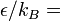$\epsilon/k_B =$ 119.8 K and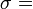$\sigma=$ 0.3405 nm). See argon for other parameter sets.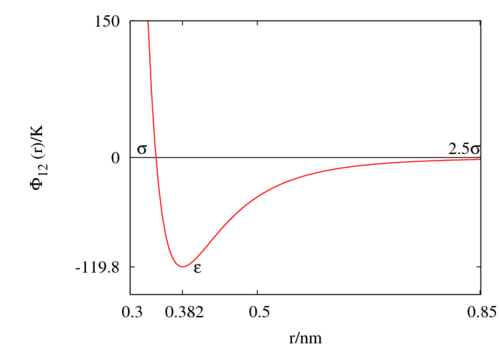## Critical point

The location of the critical point for the untruncated potential has been assessed to be at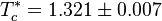$T_c^* = 1.321 \pm 0.007$

at a reduced density of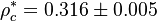$\rho_c^* = 0.316 \pm 0.005$

and the critical pressure of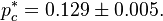$p_c^* = 0.129 \pm 0.005.$

The critical compressibility factor is given by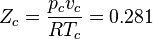$Z_c = \frac{p_cv_c}{RT_c} = 0.281$

Vliegenthart and Lekkerkerker   have suggested that the critical point is related to the second virial coefficient via the expression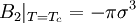$B_2 \vert_{T=T_c}= -\pi \sigma^3$

#### Truncated at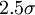$2.5 \sigma$

For the potential truncated at$2.5 \sigma$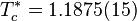$T_c^* = 1.1875 (15)$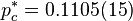$p_c^* = 0.1105 (15)$

## Triple point

The location of the triple point as found by Mastny and de Pablo  is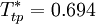$T_{tp}^* = 0.694$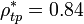$\rho_{tp}^* = 0.84$ (liquid);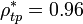$\rho_{tp}^* = 0.96$ (solid).

The following plot is of a typical radial distribution function for the monatomic Lennard-Jones liquid (here with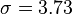$\sigma=3.73$Å and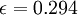$\epsilon=0.294$ kcal/mol at a temperature of 111.06K):

## Helmholtz energy function

An expression for the Helmholtz energy function of the face centred cubic solid has been given by van der Hoef , applicable within the density range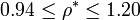$0.94 \le \rho^* \le 1.20$ and the temperature range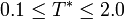$0.1 \le T^* \le 2.0$. For the liquid state see the work of Johnson, Zollweg and Gubbins .

## Vapor-liquid equilibrium

The vapor-liquid equilibrium of the Lennard-Jones potential has been studied more than 45 times in the literature. Several of these data sets were found to have gross deviations to an entity of data sets, which is both thermodynamically consistent and in good mutual agreement . The mutual agreement of these data sets was found to be approximately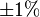$\pm 1%$ for the vapor pressure,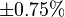$\pm 0.75%$ for the enthalpy of vaporization,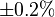$\pm 0.2%$ for the saturated liquid density, and$\pm 1%$ for the saturated vapor density .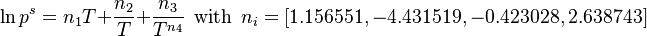$\ln p^s = n_1 T + \frac{n_2}{T} + \frac{n_3}{T^{n_4}} ~~ \mathrm{with} ~~ n_i =[ 1.156551, -4.431519, -0.423028, 2.638743 ]$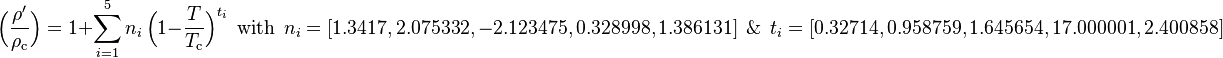$\Big(\frac{\rho'}{\rho_\mathrm{c}}\Big) = 1 + \sum_{i=1}^{5} n_i\, \Big(1 - \frac{T}{T_\mathrm{c}} \Big)^{t_i} ~~ \mathrm{with}~~ n_i = [1.3417, 2.075332, -2.123475, 0.328998, 1.386131] ~~\& ~~ t_i = [0.32714, 0.958759, 1.645654, 17.000001, 2.400858]$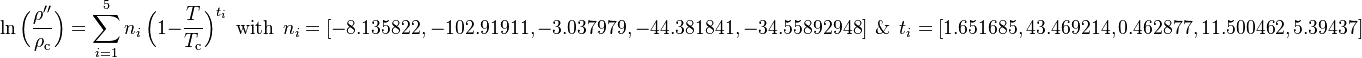$\ln \Big(\frac{\rho''}{\rho_\mathrm{c}}\Big) = \sum_{i=1}^{5} n_i\, \Big(1 - \frac{T}{T_\mathrm{c}} \Big)^{t_i} ~~ \mathrm{with}~~ n_i = [-8.135822, -102.91911, -3.037979, -44.381841, -34.55892948] ~~\& ~~ t_i = [1.651685, 43.469214, 0.462877, 11.500462, 5.39437]$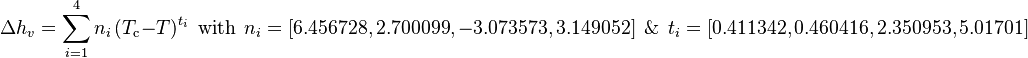$\Delta h_v = \sum_{i=1}^{4} n_i\, (T_\mathrm{c} - T)^{t_i} ~~ \mathrm{with} ~~ n_i = [6.456728, 2.700099, -3.073573, 3.149052 ] ~~\& ~~ t_i = [0.411342, 0.460416, 2.350953, 5.01701 ]$

## Melting line

The solid and liquid densities along the melting line  are given by the following equations:

#### van der Hoef

van der Hoef (Ref.  Eqs. 25 and 26):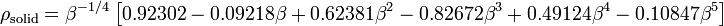$\rho_{\mathrm {solid}} = \beta^{-1/4} \left[ 0.92302 - 0.09218 \beta + 0.62381 \beta^2 -0.82672 \beta^3 + 0.49124 \beta^4 -0.10847 \beta^5\right]$

and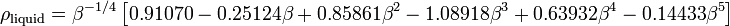$\rho_{\mathrm {liquid}} = \beta^{-1/4} \left[ 0.91070 - 0.25124 \beta + 0.85861 \beta^2 -1.08918 \beta^3 + 0.63932 \beta^4 -0.14433 \beta^5\right]$

#### Mastny and de Pablo

Mastny and de Pablo (Ref  Eqs. 20 and 21):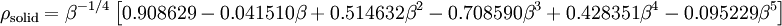$\rho_{\mathrm {solid}} = \beta^{-1/4} \left[ 0.908629 - 0.041510 \beta + 0.514632 \beta^2 -0.708590\beta^3 + 0.428351 \beta^4 -0.095229 \beta^5\right]$

and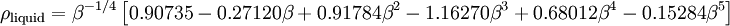$\rho_{\mathrm {liquid}} = \beta^{-1/4} \left[ 0.90735 - 0.27120 \beta + 0.91784 \beta^2 -1.16270\beta^3 + 0.68012 \beta^4 -0.15284 \beta^5\right]$

A study has been performed of the solid-fluid equilibria, and behavior in the high density region .

## Zeno line

It has been shown that the Lennard-Jones model has a straight Zeno line  on the density-temperature plane.

## Widom line

It has been shown that the Lennard-Jones model has a Widom line  on the pressure-temperature plane.

## Perturbation theory

The Lennard-Jones model is also used in perturbation theories, for example see: Weeks-Chandler-Andersen perturbation theory.

## Approximations in simulation: truncation and shifting

The Lennard-Jones model is often used with a cutoff radius of$2.5 \sigma$, beyond which$\Phi_{12}(r)$ is set to zero. Setting the well depth$\epsilon$ to be 1 in the potential on arrives at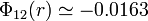$\Phi_{12}(r)\simeq -0.0163$, i.e. at this distance the potential is at less than 2% of the well depth. For an analysis of the effect of this cutoff on the melting line see the work of Mastny and de Pablo  and of Ahmed and Sadus . See Panagiotopoulos for critical parameters . See Pártay for the ground state structure . It has recently been suggested that a truncated and shifted force cutoff of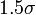$1.5 \sigma$ can be used under certain conditions . In order to avoid any discontinuity, a piecewise continuous version, known as the modified Lennard-Jones model, was developed.

## Cutoff Lennard-Jones potential

The cutoff Lennard-Jones potential is given by (Eq. 2 in ):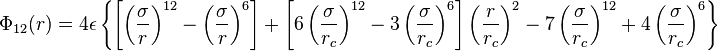$\Phi_{12}(r) = 4 \epsilon \left\{ \left[ \left(\frac{\sigma}{r} \right)^{12}- \left( \frac{\sigma}{r}\right)^6 \right]+ \left[ 6\left(\frac{\sigma}{r_c} \right)^{12}- 3\left( \frac{\sigma}{r_c}\right)^6 \right] \left(\frac{r}{r_c} \right)^2 -7 \left(\frac{\sigma}{r_c} \right)^{12} + 4 \left(\frac{\sigma}{r_c} \right)^{6} \right\}$

where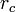$r_c$ is the cutoff radius.

## n-m Lennard-Jones potential

It is relatively common to encounter potential functions given by: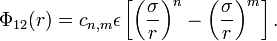$\Phi_{12}(r) = c_{n,m} \epsilon \left[ \left( \frac{ \sigma }{r } \right)^n - \left( \frac{\sigma}{r} \right)^m \right].$

with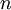$n$ and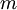$m$ being positive integers and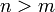$n > m$.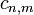$c_{n,m}$ is chosen such that the minimum value of$\Phi_{12}(r)$ being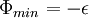$\Phi_{min} = - \epsilon$. Such forms are usually referred to as n-m Lennard-Jones Potential. For example, the 9-3 Lennard-Jones interaction potential is often used to model the interaction between a continuous solid wall and the atoms/molecules of a liquid. On the '9-3 Lennard-Jones potential' page a justification of this use is presented. Another example is the n-6 Lennard-Jones potential, where$m$ is fixed at 6, and$n$ is free to adopt a range of integer values. The potentials form part of the larger class of potentials known as the Mie potential.
Examples:

## Equation of state

Main article: Lennard-Jones equation of state

## Virial coefficients

Main article: Lennard-Jones model: virial coefficients

## Phase diagram

Main article: Phase diagram of the Lennard-Jones model

Mixtures